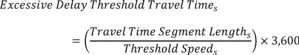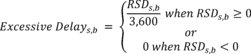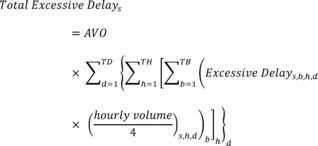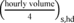Title 23

SECTION 490.711

490.711 Calculation of Peak Hour Excessive Delay metric.

§ 490.711 Calculation of Peak Hour Excessive Delay metric.

(a) The performance metric required to calculate the measure specified in § 490.707(a) is Total Peak Hour Excessive Delay (person-hours)(referred to as the PHED metric). The following paragraphs explain how to calculate this PHED metric.

(b) State DOTs shall use the following data to calculate the PHED metric:

(1) Travel times of all traffic (“all vehicles” in NPMRDS nomenclature) during each 15 minute interval for all applicable reporting segments in the travel time data set occurring for peak periods from January 1st through December 31st of the same year;

(2) The length of each applicable reporting segment, reported as required under § 490.709(b);

(3) Hourly volume estimation for all days and for all reporting segments where excessive delay is measured, as specified in § 490.709(c);

(4) Annual vehicle classification data for all days and for all reporting segments where excessive delay is measured, as specified in § 490.709(d); and

(5) Annual vehicle occupancy factors for cars, buses, and trucks for all days and for all reporting segments where excessive delay is measured, as specified in § 490.709(e).

(c) The State DOT shall calculate the “excessive delay threshold travel time” for all applicable travel time segments as follows:Where: Excessive Delay Threshold Travel Times = the time of travel, to the nearest whole second, to traverse the Travel Time Segment at which any longer measured travel times would result in excessive delay for the travel time segment “”; Travel Time Segment Lengths = total length of travel time segment to the nearest thousandth of a mile for travel time reporting segment “”; and Threshold Speeds = the speed of travel at which any slower measured speeds would result in excessive delay for travel time reporting segment “.” As defined in § 490.705, the speed threshold is 20 miles per hour (mph) or 60 percent of the posted speed limit travel time reporting segment “s,” whichever is greater.

(d) State DOTs shall determine the “excessive delay” for each 15 minute bin of each reporting segment for every hour and every day in a calendar year as follows:

(1) The travel time segment delay (RSD) shall be calculated to the nearest whole second as follow:

RSDs,b − Excessive Delay Threshold Travel Times and RSDs,b ≤ 900 seconds Where: RSDs,b = travel time segment delay, calculated to the nearest whole second, for a 15-minute bin “b” of travel time reporting segment “s” for in a day in a calendar year. RSD(s)b not to exceed 900 seconds; Travel times,b = a measured travel time, to the nearest second, for 15-minute time bin “b” recorded for travel time reporting segment “s”; Excessive Delay Threshold Travel Times = The maximum amount of time, to the nearest second, for a vehicle to traverse through travel time segment “s” before excessive delay would occur, as specified in paragraph (c) of this section; b = a 15-minute bin of a travel time reporting segment “s”; and s = a travel time reporting segment.

(2) Excessive delay, the additional amount of time to traverse a travel time segment in a 15-minute bin as compared to the time needed to traverse the travel time segment when traveling at the excessive delay travel speed threshold, shall be calculated to the nearest thousandths of an hour as follows:Where: Excessive Delays,b = excessive delay, calculated to the nearest thousandths of an hour, for 15-minute bin “b” of travel time reporting segment “s”; RSDs,b = the calculated travel time reporting segment delay for fifteen minute bin “b” of a travel time reporting segment “s,” as described in paragraph (d)(1) of this section; b = a fifteen minute bin of a travel time reporting segment “s”; and s = a travel time reporting segment.

(e) State DOTs shall use the hourly traffic volumes as described in § 490.709(c) to calculate the PHED metric for each reporting segment as follows:Where: Total Excessive Delays (in person-hours) = the sum of the excessive delay, to the nearest thousandths, for all traffic traveling through single travel time reporting segment “s” on NHS within an urbanized area, specified in § 490.703, accumulated over the full reporting year; AVO = Average Vehicle Occupancy; s = a travel time reporting segment; d = a day of the reporting year; TD = total number of days in the reporting year; h = single hour interval of the day where the first hour interval is 12 a.m. to 12:59 a.m.; TH = total number of hour intervals in day “h”; b = 15-minute bin for hour interval “h”; TB = total number of 15-minute bins where travel times are recorded in the travel time data set for hour interval “h”; Excessive Delays,b,h,d = calculated excessive travel time, in hundredths of an hour, for 15 minute bin (), hour interval (h), day (d), and travel time segment (s), as described in paragraph (d)(2) of this section; andWhere the equation equals hourly traffic volume, to the nearest tenth, for hour interval “h” and day “d” that corresponds to 15-minute bin “b” and travel time reporting segment “s” divided by 4. For example, the 9 a.m. to 9:15 a.m. minute bin would be assigned one fourth of the hourly traffic volume for the 9 a.m. to 9:59 a.m. hour on the roadway in which travel time segment is included;

AVO = (PC × AVOC) + (PB × AVOB) + (PT × AVOT)

Where: PC = the percent of cars as a share of total AADT on the segment as specified in § 490.709(d); PB = the percent of buses as a share of total AADT on the segment as specified in § 490.709(d); PT = the percent of trucks as a share of total AADT on the segment as specified in § 490.709(d); AVOC = the average vehicle occupancy of cars as specified in § 490.709(e); AVOB = the average vehicle occupancy of buses as specified in § 490.709(e); and AVOT = the average vehicle occupancy of trucks as specified in § 490.709(e).

(f) Starting in 2018 and annually thereafter, State DOTs shall report the PHED metric (to the nearest one hundredth hour) in accordance with HPMS Field Manual by June 15th of each year for the previous year's PHED measures. The PHED metric shall be reported for each reporting segment. All reporting segments of the NPMRDS shall be referenced by NPMRDS TMC or HPMS section(s). If a State DOT elects to use, in part or in whole, the equivalent data set, all reporting segments shall be referenced by HPMS sections.